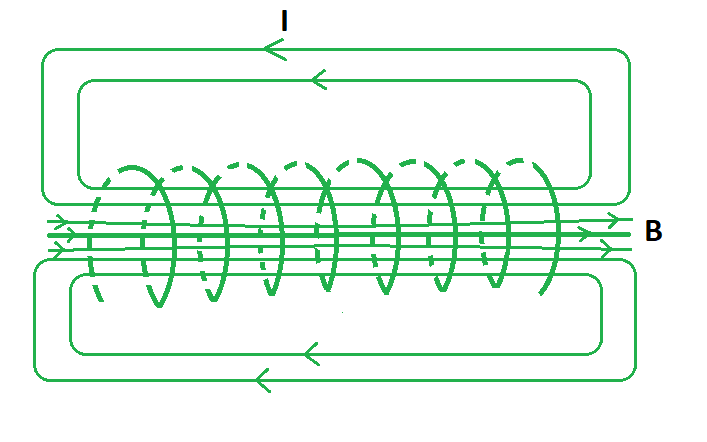# Magnetic Field In A Solenoid Formula

• Last Updated : 28 Apr, 2022

A solenoid is a long wire twisted in the shape of a helix that aids in generating a uniform magnetic field. It can be interpreted as a circular loop if the turns have less space between them. The uniformity of the internal magnetic field of a solenoid increases with an increase in its length. The total magnetic field of a solenoid is equal to the summation of magnetic fields produced at each of its turns. An ideal solenoid has zero external fields and a uniform internal field as its length is way more than the radius of the turns.### Magnetic Field In A Solenoid Formula

The magnetic field in a solenoid is maximum when the length of the solenoid is greater than the radius of its loops. It depends on various factors such as the number of turns per unit length, the current strength in the coil and permeability of the material used in the solenoid. The magnetic field of a solenoid is given by the formula:

B = μoIN/L

where,

μo is the permeability constant with a value of 1.26 × 10−6 T/m,

N is the number of turns in the solenoid,

I is the current passing through the coil,

L is the coil length.

Derivation

The formula for magnetic field inside a solenoid can be derived through Ampere’s Circuital Law.

Consider a solenoid of length n such that current I passing through each turn.

We know the integral over a closed amperian path rectangular in shape is given by,…… (1)

Now the total current that passes through the solenoid is given by,

IT = Number of turns in solenoid × Current passing through each turn

IT = (nh) (I)               …… (2)

Using Ampere’s law we can conclude that,From (1) and (2), we have

Bh = μoIT

Bh = μoI (nh)

B = μonI

Putting n = N/L as n is the number of units per unit length, we get

B = μoIN/L

This derives the formula for magnetic field inside a solenoid.

### Sample Problems

Problem 1. Find the value of the magnetic field inside a solenoid of 2 m and 100 turns per unit length if 5A of current is passing through it.

Solution:

We have,

n = 200, L = 2, I = 5

Find the number of turns using the formula n = N/L.

N = nl

= 200 (2)

= 400 turns

Using the formula for magnetic field we have,

B = μoIN/L

= 4π × 10–7 × (400/2) × 5

= 4π × 10–7 × 200 × 5

= 12.56 × 10-4 T

Problem 2. Find the value of the magnetic field inside a solenoid of 5 m and 500 turns per unit length if 10A of current is passing through it.

Solution:

We have,

n = 500, L = 5, I = 10

Find the number of turns using the formula n = N/L.

N = nl

= 500 (5)

= 2500 turns

Using the formula for magnetic field we have,

B = μoIN/L

= 4π × 10–7 × (2500/5) × 10

= 4π × 10–7 × 500 × 10

= 6.3 × 10-2 T

Problem 3. Find the value of the magnetic field inside a solenoid of 2 m and 200 turns if 3A of current is passing through it.

Solution:

We have,

N = 200, L = 2, I = 3

Using the formula for magnetic field we have,

B = μoIN/L

= 4π × 10–7 × (200/2) × 3

= 4π × 10–7 × 100 × 3

= 3.78 × 10-5 T

Problem 4. Find the value of the number of turns for a solenoid of 4 m if 2A of current is passing through it and the field is 1.25 × 10-4 T.

Solution:

We have,

B = 1.25 × 10-4, L = 4, I = 2

Using the formula for magnetic field we have,

B = μoIN/L

=> 1.25 × 10-4 = 4π × 10–7 × (N/4) × 2

=> N = (1.25 × 10-4)/(0.5 × 1.26 × 10−6)

=> N = 100

Problem 5. Find the value of the number of turns for a solenoid of 8 m if 6A of current is passing through it and the field is 1.88 × 10-4 T.

Solution:

We have,

B = 1.88 × 10-4, L = 8, I = 6

Using the formula for magnetic field we have,

B = μoIN/L

=> 1.88 × 10-4 = 4π × 10–7 × (N/8) × 6

=> N = (1.88 × 10-4)/(0.75 × 1.26 × 10−6)

=> N = 200

Problem 6. The line integral around a solenoid is 4 × 10–7 T/m. Find the net current of the solenoid.

Solution:

We have,.

It is known that,IT = (4 × 10–7)/(4π × 10–7)

= 0.31 A

Problem 7. Find the current passing through a solenoid if the number of turns is 300 for a length of 10 m and the field is 5.3 × 10-4 T.

Solution:

We have,

B = 5.3 × 10-4, L = 10, N = 300

Using the formula for magnetic field we have,

B = μoIN/L

=> 5.3 × 10-4 = 4π × 10–7 × (300/10) × I

=> I = (5.3 × 10-4)/(30 × 1.26 × 10−6)

=> I = 10 A

My Personal Notes arrow_drop_up